Question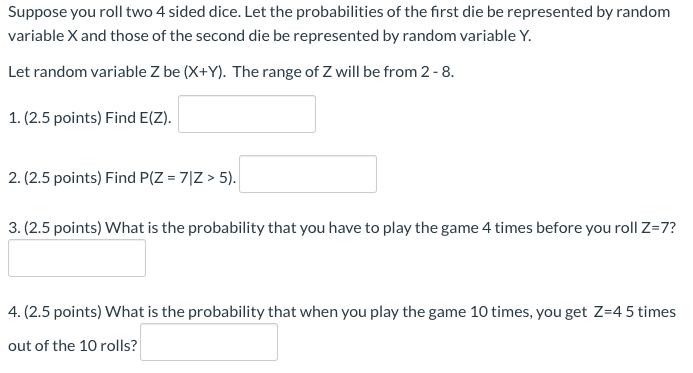The value of Z for each X, Y here is obtained as:

 X Y Z 1 1 2 1 2 3 1 3 4 1 4 5 2 1 3 2 2 4 2 3 5 2 4 6 3 1 4 3 2 5 3 3 6 3 4 7 4 1 5 4 2 6 4 3 7 4 4 8

Using this the PDF for Z here is obtained as:

 z P(z) 2 0.0625 3 0.125 4 0.1875 5 0.25 6 0.1875 7 0.125 8 0.0625

a) The expected value of Z here is computed as: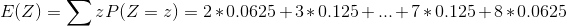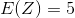Therefore 5 is the expected value here.

b) Using bayes theorem, probability here is computed as:
P(Z = 7 | Z > 5) = P(Z = 7) / P(Z = 6, 7 or 8)

= 0.125 / (0.1875 + 0.125 + 0.0625)

= 0.35

therefore 0.35 is the required probability here.

c) The probability that we will have to play 4 games before we roll Z = 7 is computed here as:

= [1 - P(Z = 7) ]4 = (1 - 0.125)4 = 0.5862

Therefore 0.5862 is the required probability here.

d) The probability that in 10 games played, we get Z = 4 five times is computed here using binomial probability function as: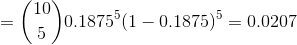Therefore 0.0207 is the required probability here.

#### Earn Coins

Coins can be redeemed for fabulous gifts.

Similar Homework Help Questions
• ### Suppose you roll two 4 sided dice. Let the probabilities of the first die be represented...Suppose you roll two 4 sided dice. Let the probabilities of the first die be represented by random variable X and those of the second die be represented by random variable Y. Let random variable Z be (X+Y). The range of Z will be from 2-8. 1.(2.5 points) Find E(Z). 2. (2.5 points) Find P(Z = 71Z > 5). 3.(2.5 points) What is the probability that you have to play the game 4 times before you roll Z=7? 4. (2.5...

• ### Suppose you roll two 4 sided dice. Let the probabilities of the first die be represented...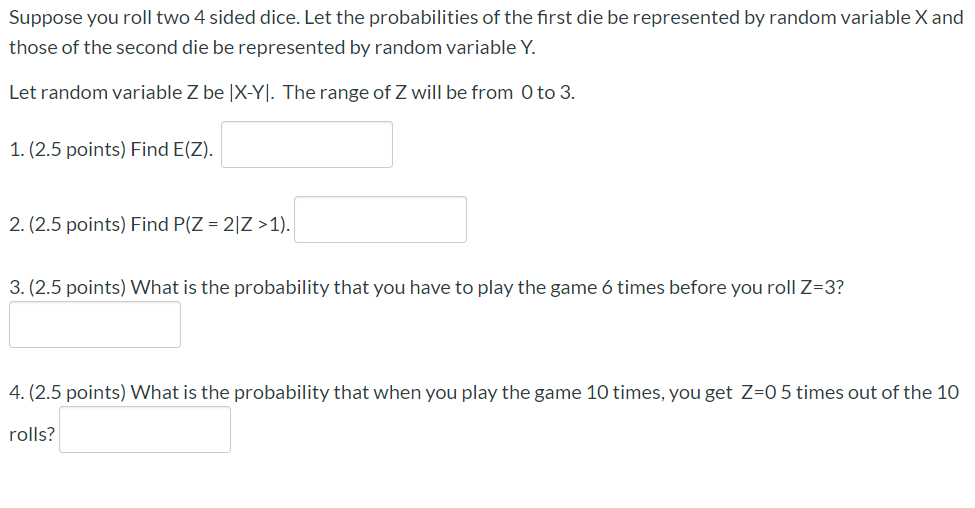Suppose you roll two 4 sided dice. Let the probabilities of the first die be represented by random variable X and those of the second die be represented by random variable Y. Let random variable Z be X-Y). The range of Z will be from 0 to 3. 1. (2.5 points) Find E(Z). 2. (2.5 points) Find P(Z = 21Z >1). 3. (2.5 points) What is the probability that you have to play the game 6 times before you roll...

• ### Suppose you roll two 4 sided dice. Let the probabilities of the first die be represented...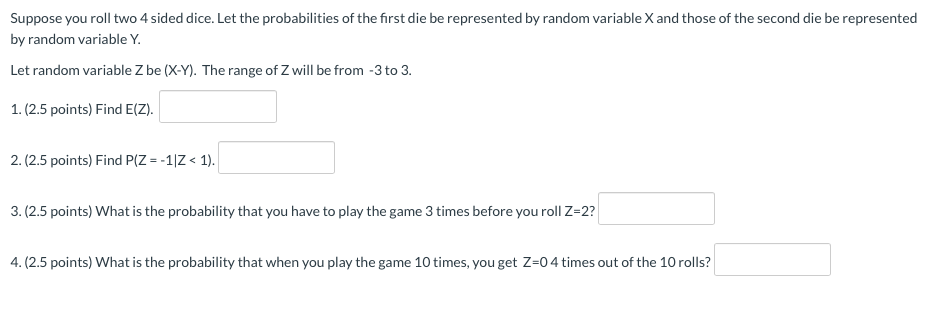Suppose you roll two 4 sided dice. Let the probabilities of the first die be represented by random variable X and those of the second die be represented by random variable Y. Let random variable Z be (X-Y). The range of Z will be from -3 to 3. 1. (2.5 points) Find E(Z). 2. (2.5 points) Find P(Z =-1|Z < 1). 3. (2.5 points) What is the probability that you have to play the game 3 times before you roll...

• ### Suppose you roll two 4 sided dice. Let the probabilities of the first die be represented...Suppose you roll two 4 sided dice. Let the probabilities of the first die be represented by random variable X and those of the second die be represented by random variable Y. Let random variable Z be (X-Y). The range of Z will be from -3 to 3. 1. (2.5 points) Find E(Z). 2. (2.5 points) Find P(Z =-1|Z < 1). 3. (2.5 points) What is the probability that you have to play the game 3 times before you roll...

• ### Suppose you roll two 4 sided dice. Let the probabilities of the first die be represented...

Suppose you roll two 4 sided dice. Let the probabilities of the first die be represented by random variable X and those of the second die be represented by random variable Y. Let random variable Z be |X-Y|. The range of Z will be from 0 to 3. 1. Find E(Z). 2. Find P(Z = 1|Z <3). 3. What is the probability that you have to play the game 4 times before you roll Z=3? 4. What is the probability...

• ### dice is unbiased. Throws independent. Step 1. You roll a six-sided die. Let X be the...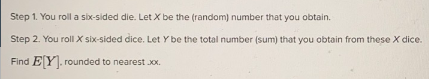dice is unbiased. Throws independent. Step 1. You roll a six-sided die. Let X be the (random) number that you obtain. Step 2. You roll X six-sided dice. Let Y be the total number (sum) that you obtain from these X dice. Find E[Y] rounded to nearest .xx.

• ### Step 1. You roll a six-sided die. Let X be the (random) number that you obtain....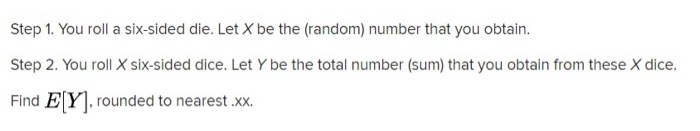Step 1. You roll a six-sided die. Let X be the (random) number that you obtain. Step 2. You roll X six-sided dice. Let Y be the total number (sum) that you obtain from these X dice. Find E[Y], rounded to nearest .XX.

• ### There exists 3-sided dice. Such a die, when you roll it, will show 1, 2, or...

There exists 3-sided dice. Such a die, when you roll it, will show 1, 2, or 3 with equal probability. The experiment is to roll 3 such dice. Random variable T is the total of all 3 dice. t P(T=t) 3 4 5 6 7 8 9

• ### 2. (25 points) Sekora International Casino (SIC) is launching a new game making use of fair 6-sided dice . In phase 1, roll two 6-sided dice and compute the difference between the rolls. Call thi...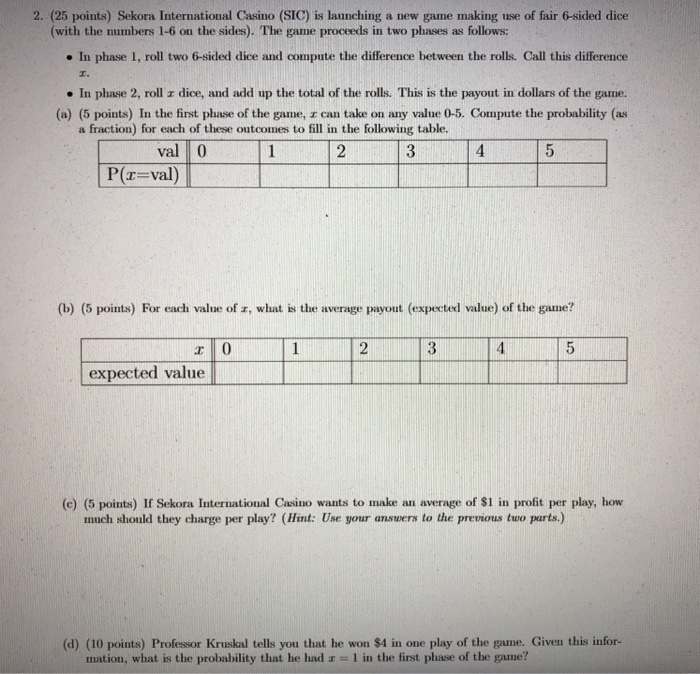2. (25 points) Sekora International Casino (SIC) is launching a new game making use of fair 6-sided dice . In phase 1, roll two 6-sided dice and compute the difference between the rolls. Call this difference . In phase 2, roll r dice, and add up the total of the rolls. This is the payout in dollars of the game. (with the numbers 1-6 on the sides). The game proceeds in two phases as follows: (a) (5 points) In the...

• ### The Dice game of "Pig" can be played with the following rules. 1. Roll two six-sided dice. Add the face values...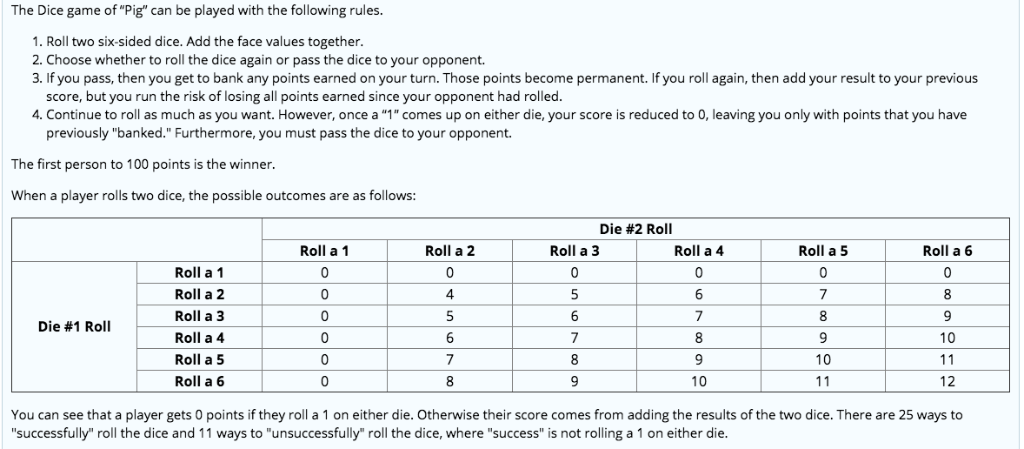The Dice game of "Pig" can be played with the following rules. 1. Roll two six-sided dice. Add the face values together. 2. Choose whether to roll the dice again or pass the dice to your opponent. 3. If you pass, then you get to bank any points earned on your turn. Those points become permanent. If you roll again, then add your result to your previous score, but you run the risk of losing all points earned since your...